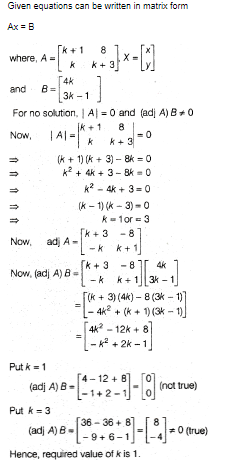# The number of values of k, for which the system of equations (k + 1)x + 8y = 4k kx + (k + 3)y = 3k - 1 has no solution, is ?Saurabh Koranglekar
2 years ago2 years ago

For solution to have infinite solutions, ratio of x coefficients=ratio of y coefficients=ratio of constants

=>(k+1)/k=8/(k+3)=4k/(3k-1)

solve (k+1)/k=8/(k+3)

=>k^2+4k+3=8k

=>k^2–4k+3=0

=>k=1,3

Now,8/(k+3)=4k/(3k-1)

=>6k-2=k^2+3k

=>k^2–3k+2=0

=>k=1,2